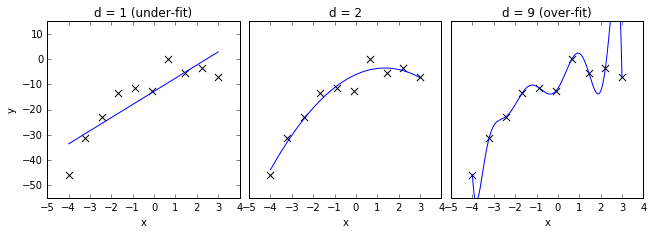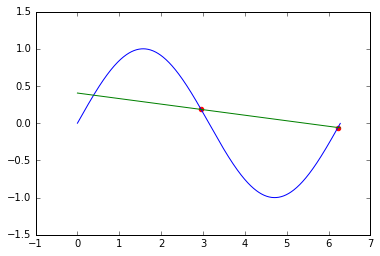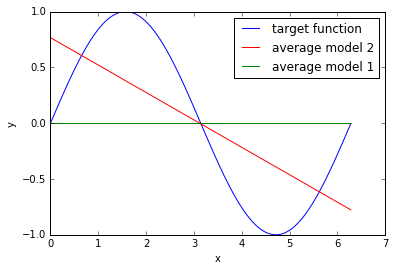## Bias Variance Tradeoff¶

### Model complexity and generalization¶

#### Learning Objectives¶

Concepts of machine learning

• Target function
• Overfitting, underfitting
• Generalization
• Out-of-sample error and test data
• Model complexity

### Target function and noise¶

For prediction: We want to approximate an unknown function, the target function $t(\vec x)$.

If the targets $y$ would be deterministically determined from the $\vec x$ values:

$$y = t(\vec {x})$$

But $y$ is typically noisy. We can model this by:

$$y^{(i)} = t(\vec {x}^{(i)}) + \epsilon$$

with a random variable $\epsilon$ (= stochastic noise)

Sidenote:

Instead of a function we now a a (conditional) probability distribution: $$p(y \mid \vec {x})$$

The probability distribution of the noise can depend on $\vec {x}$: $p(\epsilon \mid \vec {x})$.

Goal:

We are looking for "good" hypotheses. $$h(\vec x) \approx t(\vec x)$$ for all $\vec{x}$ of interest, i.e. for all possible $\vec{x}$ we want to predict. ($p(\vec x)$ suffient large).

Training data is noisy: $y^{(i)} = t(\vec {x}^{(i)}) + \epsilon$

Therefore $h(\vec{x}^{(i)})$ should not perfectly match $y^{(i)}$ of the training data.
But how good should be the correspondence?

#### Sampling Error¶

• Which pattern are caused by the target function?
• Which pattern are caused by the (random chosen) train data?

### Overfitting and Underfitting¶

In :
import numpy as np
import matplotlib.pyplot as plt
%matplotlib inline

In :
import numpy as np

def test_func(f, x, err=1.):
return np.random.normal(f(x), err)

def compute_error(x, y, p):
yfit = np.polyval(p, x)
return np.sqrt(np.mean((y - yfit) ** 2))

def plot_under_overfit_polynoms():
N = 10
np.random.seed(45)
x = np.linspace(-4, 3., N)
quadratic_func = lambda x: -2 + 3. * x + -2. * x**2
y = test_func(quadratic_func,x, 4.)

xfit = np.linspace(-4, 3., 1000)

degrees = [1, 2, 9]
titles = ['d = 1 (under-fit)', 'd = 2', 'd = '+ str(degrees) + ' (over-fit)']

plt.figure(figsize = (9, 3.5))
plt.subplots_adjust(left = 0.06, right=0.98,
bottom=0.15, top=0.85,
wspace=0.05)
yticks = [-50,-40,-30,-20,-10, 0, 10]
for i, d in enumerate(degrees):
plt.subplot(131 + i, yticks=yticks)
yticks=[]
plt.scatter(x, y, marker='x', c='k', s=50)

p = np.polyfit(x, y, d)
yfit = np.polyval(p, xfit)
plt.plot(xfit, yfit, '-b')

#pl.xlim(-0.2, 1.2)
plt.ylim(-55, 15)
plt.xlabel(u'x')
if i == 0:
plt.ylabel('y')
plt.title(titles[i])


#### Example: Linear Regression with polynominal basis functions¶

In :
plot_under_overfit_polynoms()#### Example: Classification Underfitting¶#### Example: Classification Overfitting¶#### Example: Just Right¶#### Goal of learning - Hypothesis Set $\mathcal{H}$}¶

Goal of learning: We are looking for a appropriate hypothesis $$h(\vec x) \approx t(\vec x)$$

$h$ is determined from the hypothesis set $\mathcal{H}$ by training.

Example Univariate Linear Regression'':

$\mathcal H$ is the set of all (infinit) hypotheses: $h_\theta(x) = \theta_0 + \theta_1 x$ (by variation of $\theta_0$ and $\theta_1$).

From the set $\mathcal{H}$ we get through training a $\theta$, i.e. a hypothesis $h_{\theta_{final}}({x})$.

$h_{\theta_{final}}({\bf x})$ is given by the minimum of the cost function:

$\forall \theta: J(\theta_{final}) \leq J(\theta)$

#### Underfitting¶

Recap: Goal is $h(\vec x) \approx t(\vec x)$.

What happens in the underfitting case?

The goal can not be reached: No hypothesis of $\mathcal{H}$ is similar enough to $t(\vec x)$.

The hypothesis set $\mathcal{H}$ has not suffient complexity.

#### Overfitting¶

Recap: Goal is $h(\vec x) \approx t(\vec x)$.

What happens in the overfitting case?

The trained hypothesis $h_{\theta_{final}}({x})$ is adaped to much on the training data: also on the noise pattern.

The hypothesis set $\mathcal{H}$ has to much complexity.

### Test data¶

#### Training error¶

Up to now only a training set $\mathcal{D}_{train}$ and calculation of the average loss of the traing data (cost function):

Training error (in-sample error $E_{in}(h)$):

$$E_{in}(h) = \frac{1}{m} \sum_{i=0}^{m} loss(h(\vec x^{(i)}), y^{(i)})$$

Note: We consider $E_{in}(h)$ now as a function of possible hypotheses $h$. We want to compare different models.

The low training error is not suffient to judge a "good" model.

Overfitting!

$h(\vec x)$ can make bad predictions for $\vec x$ values not in the training set.

Bad generalization.

#### Out-of-sample error¶

The out-of-sample error is the average loss of typical (new) data ($p({\vec x}', y')$ suffient large):

$$E_{out}(h) = \mathbb{E}_{\bf x,y}\left[loss(h({\vec x}), y)\right] = \int\limits_{\mathcal{X \times Y}} loss(h({\vec x}), y) dp({\vec x},y)$$

#### Generalization error¶

The generalization error of a hypothesis $h$ can defined as (after [Abu]):

$$| E_{out}(h) - E_{in}(h)|$$

#### Test data¶

Problem: We can compute the out-of-sample error $E_{out}$ directly.

Take $m_{test}$ labeled test data $\mathcal{D}_{test}$ to check if $h(\vec x)$ predicts good values even for $\vec x$ values not used in training.

$$\mathcal{D}_{test}= \{ ( {\vec {x'}}^{(0)}, {y'}^{(0)} ), ( {\vec {x'}}^{(1)}, {y'}^{(1)} ), \dots, ( {\vec {x'}}^{(m_{test})}, {y'}^{(m_{test})} ) \}$$

#### Test error¶

The test error is the average loss of the test data:

$$testerror = \frac{1}{m'} \sum_{i=0}^{m_{test}} loss(h({\vec {x'}}^{(i)}), {y'}^{(i)})$$

The test error is an approximation of the out-of-sample error $E_{out}(h)$:
$$testerror \approx \mathbb{E}_{\mathcal{X,Y}}[loss(h({\bf x}), y)] = E_{out}(h)$$

#### Model complexity, Capacity¶

informal:

The complexity of $\mathcal{H}$ describes how many compex functions the hypothesis set $\mathcal{H}$ can cover.

Measure of model complexity are

• VC-Dimension $d_{vc}$ (Vapnik–Chervonenkis Dimension)
• Rademacher complexity

#### Expectation of out-of-sample error¶

Starting point for the Bias-Variance Analysis is the expectation of out-of-sample error:

$$\mathbb{E}_{\mathcal{D}} \left[ E_{out}(h^{\mathcal{D}}({\bf x})) \right]$$

with

• $h^{\mathcal{D}}$: trained hypothesis from $\mathcal{D}$ (training data)
• $E_{out}(h^{\mathcal{D}})$: out-of-sample error for the trained hypothesis
• $\mathbb{E}_{\mathcal{D}}$: Expectation with respect to the training data (a training set is an event).

#### Bias-variance analysis¶

without noise and squared error: \begin{align*} \mathbb{E}_{\mathcal{D}} \left[ E_{out}(h^{\mathcal{D}}) \right] & = \mathbb{E}_{\mathcal{D}} \left[ \mathbb{E}_{\bf x,y} \left[ \left(h^{\mathcal{D}}({\bf x}) - t({\bf x})\right)^2 \right] \right] \\ & = \mathbb{E}_{\bf x,y} \left[ \mathbb{E}_{\mathcal{D}} \left[ \left(h^{\mathcal{D}}({\bf x}) - t({\bf x})\right)^2 \right] \right] \end{align*}

with the average hypothesis $$\tilde{h}({\bf x}) = \mathbb{E}_{\mathcal{D}}\left[ h^{\mathcal{D}}({\bf x}) \right]$$

We get (see e.g. [Abu] p.63): \begin{align*} \mathbb{E}_{\mathcal{D}} \left[ E_{out}(h^{\mathcal{D}}({\bf x})) \right] &= \mathbb{E}_{\bf x,y} \left[ \mathbb{E}_{\mathcal{D}} \left[\left(h^{\mathcal{D}}({\bf x}) - \tilde{h}({\bf x}) \right)^2\right]\right] &+&\mathbb{E}_{\bf x,y} \left[ \left(\tilde{h}({\bf x}) -t({\bf x}) \right)^2 \right]& \\ &= variance &+&bias^2 \end{align*}

with noisy data there is a thrid term: the irreducable error $\mathbb{E}_{\mathcal X}[\epsilon^2]$ (Bayes Error, see e.g. [Has])}

#### Interpretation: Bias¶

$$\mathbb{E}_{\bf x,y} \left[ \left(\tilde{h}({\bf x}) -t({\bf x}) \right)^2 \right]$$ Quadratic deviation of the average hypothesis $\tilde h$ from the target function $t$ $\leftrightarrow$ Underfitting#### Interpretation: Variance¶

$$\mathbb{E}_{\bf x,y} \left[ \mathbb{E}_{\mathcal{D}} \left[\left(h^{\mathcal{D}}({\bf x}) - \tilde{h}({\bf x}) \right)^2\right]\right]$$

Average quadratic deviation {$h^{\mathcal{D}}$} from the average hyposesis $\tilde h$ $\leftrightarrow$ Overfitting#### Example¶

from [Abu]

• Target function $sin(x)$
• $p(x)$: Uniform distributed in the range $(0, 2 \pi)$
• no noise
• only two training examples. i.e. $n=2$
• Model 1: $h(x)=w$ (constant)
• Model 2: $h(x)=\theta_0 + \theta_1 x$

#### Exercise¶

Approximate: $\mathbb E (E_{out})$, the bias and the variance for both models by sampling. A sample correspond to a training set (with 2 examples)

In :
x, y  = train_data()
theta_0, theta_1 = get_theta(x, y)
plt.plot(x_, y_, 'b-')
plt.scatter(x,y,color='r')
plt.plot(x_,  theta_0 + theta_1 * x_, 'g-')

Out:
[<matplotlib.lines.Line2D at 0x10ef89358>]No noise on $y$ : $$\mathbb E_{\bf x, \bf y} [ E_{out} ] = \int_{-\infty}^{\infty} (t(x)- h(x))^2 p(x) dx = \int_{0}^{2 \pi} (t(x)- h(x))^2 dx$$

In :
expectation_Eout_1 = e_out_m1.mean()
print ("expectation of E_out of model 1:", expectation_Eout_1)

expectation of E_out of model 1: 0.7495649709872853

In :
expectation_Eout_2 = e_out_m2.mean()
print ("expectation of E_out of model 2:", expectation_Eout_2)

expectation of E_out of model 2: 1.8852093027834775

In :
# Average Model 1 and 2
plt.plot(x_, y_, 'b-', label='target function')
plt.plot(x_,  avg_theta_0 + avg_theta_1 * x_, 'r-', label='average model 2')
plt.plot(x_,  np.ones(len(x_)) * avg_w, 'g-', label='average model 1')
plt.xlabel("x"); plt.ylabel("y"), plt.legend()

Out:
(<matplotlib.text.Text at 0x10efc5438>,
<matplotlib.legend.Legend at 0x10f4379e8>)From the plot we see that the bias of model 2 is smaller than the bias of model 1.

In :
print ("Bias of model 1:", bias_1)

Bias of model 1: 0.4994622029361333

In :
print ("Bias of model 2:", bias_2)

Bias of model 2: 0.20764158671729008

In :
print ("Variance of model 1:", variance_1)

Variance of model 1: 0.2502570759559479

In :
print ("Variance of model 2:", variance_2)

Variance of model 2: 1.6858020395241051

In :
print("model 1: E_out ≈ bias + variance:  %f ≈ %f + %f" % (expectation_Eout_1, bias_1, variance_1))
print("model 2: E_out ≈ bias + variance:  %f ≈ %f + %f" % (expectation_Eout_2, bias_2, variance_2))

model 1: E_out ≈ bias + variance:  0.749565 ≈ 0.499462 + 0.250257
model 2: E_out ≈ bias + variance:  1.885209 ≈ 0.207642 + 1.685802


### Learning curves¶

#### Goal¶

$$h(\vec x) \approx t(\vec x)$$ reached if $E_{out}$ is small (small test error)

Both conditions must be satisfied

• $E_{in}$ small training error (low bias, no underfitting)
• Small generalization error $|E_{out} -E_{in}|$ respectively $test error \approx trainings error$ (low variance, no overfitting)

How can we know that the model is adequate?

Adequate in respect to:

• Number of training data $m$ of $\mathcal{D}_{train}$
• Model complexity $\mathcal{H}$
• Signal-noise ration: strength of stochastic noise $\epsilon$ in respect to the target function $t$

#### Learning curve of a simple model¶#### Learning curve of a complex model¶